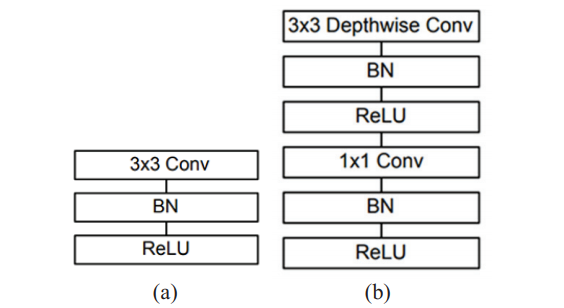# What is Object Detection?

Object detection is the process of finding instances of real-world objects such as faces, bicycles, and buildings in images or videos. Object detection algorithms typically use extracted features and learning algorithms to recognize instances of an object category. It is commonly used in applications such as image retrieval, security, surveillance, and automated vehicle parking systems.

# Single Shot Detector (SSD)

The SSD object detection composes of 2 parts:

1. Extract feature maps: It extracts the features presented in the image. A feature map is the output of CNN which will extract some important portion in the image.
2. Apply convolution filters to detect objects: It will classify the object present in the image and build the bounding boxes around them.

There are two Models: SSD300 and SSD512:

• SSD300: 300×300 input image, lower resolution, faster.
• SSD512: 512×512 input image, higher resolution, more accurate.

It is significantly faster in speed and high-accuracy object detection algorithm. A quick comparison between speed and accuracy of different object detection models on VOC2007:

• SDD300: 59 FPS with mAP 74.3%
• SSD512: 22FPS with mAP 76.9%
• Faster R-CNN: 7 FPS with mAP 73.2%
• YOLO: 45 FPS with mAP 63.4%

# MobileNet

MobileNet is a CNN architecture model for Image Classification and Mobile Vision. There are other models as well but what makes MobileNet special is that it has very little computation power to run or apply transfer learning. This makes it a perfect fit for Mobile devices, embedded systems, and computers without GPU or low computational efficiency with compromising significantly with the accuracy of the results.

MobileNet uses depthwise separable convolutions. It significantly reduces the number of parameters when compared to the network with regular convolutions with the same depth in the nets. This results in lightweight deep neural networks.(a) Standard convolutional layer with batch normalization and ReLU. (b) Depth-wise separable convolution with depth-wise and pointwise layers followed by batch normalization and ReLU.

A depthwise separable convolution is made from two operations:

1. Depthwise convolution
2. Pointwise convolution

It is a map of a single convolution on each input channel separately. Therefore its number of output channels is the same as the number of the input channels. Its computational cost is Df² * M * Dk².

Convolution with a kernel size of 1x1 that simply combines the features created by the depthwise convolution. Its computational cost is M * N * Df².

If we combine both the MobileNet architecture and the Single Shot Detector (SSD) framework, we arrive at a fast, efficient deep learning-based method to object detection.

The model we’ll be using in this blog post is a Caffe version of the original TensorFlow implementation by Howard et al. and was trained by chuanqi305 (see GitHub). The MobileNet SSD was first trained on the COCO dataset (Common Objects in Context).

MobileNet SSD + deep neural network (dnn) module in OpenCV to build object detector

# Code Implementation

Importing libraries

`import cv2import numpy as np`

Loading the MobileNet SSD model and deploying the weights and initializing the video stream. Reading input frames, resizing, and extracting dimensions of the frame.

`thres = 0.45nms_threshold = 0.2cap = cv2.VideoCapture(0)cap.set(3,640)cap.set(4,480)cap.set(10,150)classNames = []classFile = 'coco.names'with open(classFile,'rt') as f:    classNames = f.read().rstrip('\n').split('\n')print(classNames)configPath = 'ssd_mobilenet_v3_large_coco_2020_01_14.pbtxt'weightsPath = 'frozen_inference_graph.pb'net = cv2.dnn_DetectionModel(weightsPath,configPath)net.setInputSize(320,320)net.setInputScale(1.0/ 127.5)net.setInputMean((127.5, 127.5, 127.5))net.setInputSwapRB(True)`

Looping over each detection and storing confidence- prediction percentage of each object corresponding to each label. Filtering out weak detections and storing the index ID of each object. Extract the localized coordinates of each object. Drawing a bounding box over the detected object along with label and confidence percentage. Displaying live streaming with detections and bounding boxes and an escape command.

`while True: success,img = cap.read() classId, confs, bbox = net.detect(img,confThreshold= thres) bbox = list(bbox) confs = list(np.array(confs).reshape(1, -1)) confs = list(map(float,confs)) print(type(confs)) print(confs)  indices = cv2.dnn.NMSBoxes(bbox,confs,thres,nms_threshold) print(indices) for i in indices:     i = i     box = bbox[i]     x,y,w,h = box,box,box,box     cv2.rectangle(img, (x,y),(x+w,h+y), color=(0, 255, 0),      thickness=2)     cv2.putText(img, classNames[classId [i]-1].upper(), (box + 10, box + 30), cv2.FONT_HERSHEY_COMPLEX,1,(0,255,0),2) cv2.imshow("Object Detection", img) key = cv2.waitKey(1) & 0xFF if key == ord("q"):     breakcv2.VideoCapture.release()cv2.destroyAllWindows()`

SSD Framework through faster than other similar alternatives finds trouble while detecting smaller objects (still performing better than YOLO).

Apart from SSD, other frameworks can be implemented in object detection, the more popular ones being YOLO and Fast/Faster-R CNN. The three have their own set of pros and cons, however, the SSD method is the fastest and most efficient among these. To learn more about YOLO and its various versions read here.